# Analysis of report measuring body, weight, and metabolic rate

Professor Mike Veatch

MAT220B Biostatistics: Kinesiology project

Introduction

The purpose of this study is to evaluate the validity of a study involving men, women, weight, and metabolic rate. I will calculate regression of the relationships between these variables. I will also ask what type of relationship these variables have, linear, quadratic? Also, what is the best type of equation to model these variables with, one with multiple or a single variable?

Data Collection

Metabolic rate is a measurement that reports kilocalories burned per 24 hours.  This can be measured with a few different methods; the most accurate is indirect calorimetry.  Easier, but less accurate is the use of a device such as the BodyGem, and the easiest of all is to use an equation, such as the Weir equation. 

1.

The results may be affected by the fact that the subjects were dieting. Certain subjects may shift in metabolic rate as a reaction to the changed diet more than other subjects. This could create lower correlation in data by having a spread of subjects, some of which fluctuate more than others in metabolic rate as a reaction the changed diet.

2.

The regression lines for the males has a lower slope than the female regression line, meaning that the male rate is greater than the female rate at low body mass (Fig. 1). The male average rate is greater than the female average rate. The greater y-intercept could be because males have a greater baseline metabolic rate, while the greater slope associated with the female regression line slope could be attributed to their small body mass that as it increases, the rate needs to greatly adjust to compensate for their smaller initial metabolic rate. The correlation for females is much stronger than for males (.768 female vs. .351 male). This could be because there is greater variation in male metabolic rate, which assumes good data collection.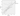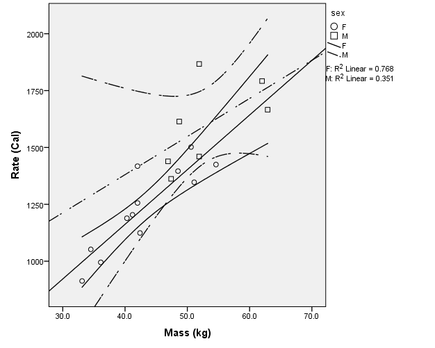Figure 1. Scatterplot of mass (explanatory) vs. metabolic rate (response). 95% confidence intervals included.

3.

The three conditions for inference are normal residuals, linearity, and constant standard deviation.  There is a violation of constant standard deviation, because the spread of points starts small, but becomes larger as y values increase. Linearity is not violated, however, there may be slight concave down curve for the male relationship between mass and metabolic rate. More points exist below the line of regression than above, and if I imagine a line slightly curved concave down a better fit may be found for this data. Based on the same logic, there does not seem to be a curve in the line for the female relationship, because the spread of points seems evenly distributed above and below the line of regression. There is not a violation of normal residuals, because if a y = 0 line is drawn, a line with no slope that originates at y = 0, about half of the points fall on either side of this line, making it a normal distribution. The point at the top right of the graph may be an outlier, but if the regression line of this chart has a positive slope, it may not be too far from the regression line; its residual may not be much greater than the average residual length.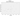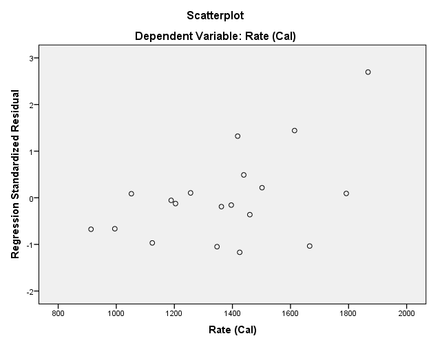Figure 2. Rate (explanatory variable) vs. regression standardized residual for a regression between rate and mass.

4.

H0 = there is no correlation between mass and rate. Ha = there is a correlation between mass and rate. Rate and sex do have a relationship, and so do rate and mass, because both of them have significance values below the alpha level of 0.1.  The equation of this multiple regression is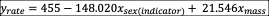. For the indicator variable, female = 1, and male = 0. Using this formula, we can calculate the mean rate for a 45kg female: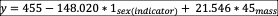, yields y = 1276.55. The p value is below 0.05 ( p < 0.000 ), therefore we conclude that there is significant correlation between these variables.

5.

The R2 from a regression involving mass and sex as explanatory variables and rate as a response variable is 0.799. The R2 between mass and rate yields an R2 of 0.748. The R2 between sex and rate is 0.493. The R2 between interaction and rate is 0.312. Therefore, the model including mass and sex as explanatory variables gives the greatest correlation. All three of these tests have significance levels below 0.1, so a linear relationship models all of these variables well. However, the R2 of the interaction as explanatory variable is too low (0.312) to make for a decent model.

6.

In figure 1, I present a scatterplot of weight (explanatory) vs. rate (response) in which there is graphed onto the plot a confidence interval (CI) of 95%. These confidence intervals tell us that we can predict with 95% confidence that the mean rate is between the CI lines. The CI for females is more accurate—more narrow than the CI for males.

Conclusions

I found significant relationships between all of the variables, as evidenced by the P values yielded by the regression tests I performed. The regression with mass and sex as explanatory variables has a p value less than 0.000, with interaction as an explanatory variable gives a p value of 0.013, of mass only yields less than 0.000, and of sex equals 0.001.

# Works Cited

Brigitte Baldi, D. S. (2012). The Practice of Statistics in the LIfe SCiences. New York, NY: W.H. Freeman and Company.

Kelly, M. P. (2014). Resting Metabolic Rate: Best Ways to Measure It—And Raise It, Too. Retrieved April 25, 2014, from American Council on Exercise: http://www.acefitness.org/certifiednewsarticle/2882/resting-metabolic-rate-best-ways-to-measure-it-and/

 (Brigitte Baldi 73 – 74, 2012)

 (Kelly, 2014)

 (Brigitte Baldi 582 – 583, 2012)

 (Brigitte Baldi 28-8 – 28-12, 2012)

Categorization:
Biology
,
Mathematics